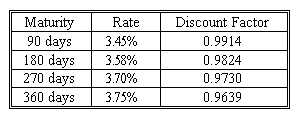### CFA Practice Question

There are 227 practice questions for this study session.

### CFA Practice Question

Consider a European payer swaption that expires in two years and is exercisable as a one-year swap with quarterly payments, using 90/360 as the day-count adjustment. The exercise rate is 3.6%. The notional principal is \$20 million. Now suppose we are at the swaption expiration and the term structure is as follows:What is the value of the swaption right before expiration?
A. \$15,643
B. \$4,000
C. \$184,000
Explanation: Under these conditions, the swap fixed payment should be 0.0092, equating to an annual fixed rate of 3.68%. The holder of the swaption has the right to enter into a swap to pay 3.6% (which corresponds to a quarterly payment of \$180,000), whereas in the market such a swap would require payment at a rate of 3.68% (which corresponds to a quarterly payment of \$184,000). If the holder exercises the swap, and enters an opposite swap in the market, he effectively receives a net quarterly payment stream of \$184,000 - \$180,000 = \$4,000. The present value of this payment stream is \$4,000 (0.9914 + 0.9824 + 0.9730 + 0.9639) = \$15,643.

User Comment
danlan2 Where does 3.68% come from?
danlan2 3.68%=sum of all discount factor
=0.9914+0.9824+0.9730+0.9639
frankal101 no it does not...
ThePessimist (360/90)*(1-0.9639)/(sum of discount factors)=3.68%
ReeM 1-Z4/(Z1+Z2+Z3+Z4)
Doing what you said results in 3.6924%.
(360/90)*(1-0.9639)/(.9914+.9824+.973+.9639)=3.6924%
actually carry out the calculations and you will see, 3.68% is a mystery
DZ2008 Ahhh I see ... they rounded to 0.0092 for this part: (1-0.9639)/(.9914+.9824+.973+.9639) even though it should actually be 0.009231, which makes a big difference
dblueroom Yep, if you had 3.6924, you would end up with 17,000 + I used 3.069%
HectorRS2 Rat diff: 3.68% - 3.60%=.08%
Annualized to quaterly: .08% * 90/360 = .02%
Quaterly Payment: .02% * 20.000.000= 4.000
PV =4.000*(discount factors) =15.643
uviolet ThePessimist is correct. Use the Fixed rate formula and then multiply it by 4 to get the annual rate of 3.68